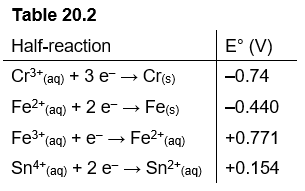# Problem: Which of the following reactions will occur spontaneously as written?A) Sn4+ (aq) + Fe2+ (aq) → Sn2+ (aq) + Fe (s) B) 3 Fe (s) + 2 Cr3+ (aq) → 2 Cr (s) + 3Fe2+ (aq)C) 3 Fe2+ (aq) → Fe (s) + 2 Fe3+ (aq)D) 3 Sn4+ (aq) + 2 Cr (s) → 2 Cr3+ (aq) + 3 Sn2+ (aq)E) Sn4+ (aq) + Fe3+ (aq) → Sn2+ (aq) + Fe2+ (aq)

###### FREE Expert Solution

We’re being asked if the given redox reaction occurs spontaneously in the forward direction.

Reaction:      2 Al(s) + 3 Pb2+(aq) → 2 Al3+(aq) + 3 Pb(s)

Recall that the greater the E°cell of a redox reaction, the more likely the reaction will occur (more spontaneous reaction)

E°cell > 0 spontaneous in the forward direction
E°cell < 0 nonspontaneous in the forward direction

We will have to calculate for the cell potential to determine the spontaneity of the redox reaction using the following steps:

81% (347 ratings)###### Problem DetailsWhich of the following reactions will occur spontaneously as written?

A) Sn4+ (aq) + Fe2+ (aq) → Sn2+ (aq) + Fe (s)

B) 3 Fe (s) + 2 Cr3+ (aq) → 2 Cr (s) + 3Fe2+ (aq)

C) 3 Fe2+ (aq) → Fe (s) + 2 Fe3+ (aq)

D) 3 Sn4+ (aq) + 2 Cr (s) → 2 Cr3+ (aq) + 3 Sn2+ (aq)

E) Sn4+ (aq) + Fe3+ (aq) → Sn2+ (aq) + Fe2+ (aq)